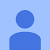# Understanding Clustering

## Importance of Clustering :

Clustering is the process of grouping data items that are “similar” between them, and “dissimilar” to data items in other clusters.

Clustering separates datasets into many clusters of similar ones and finding out grouping in data automatically.

So, the main purpose of clustering is to separate groups with similar behaviors and combine them together into diverse clusters.

It is very challenging for a machine to recognize from an orange or an apple unless as it should be trained on a huge amount of relevant dataset. This training is done by using unsupervised machine learning algorithms, such as clustering.

For example…

# Concepts of Linear Regression, Bias-Variance Tradeoff, and Regularisation

## What is Regression?

Before learning about linear regression, let us get ourselves accustomed to regression. Regression is a method of modeling a target value based on independent predictors. It is a statistical tool which is used to find out the relationship between the outcome variable also known as the dependent variable, and one or more variable often called as independent variables.

## When and why do you use Regression?

Regression is performed when the dependent variable is of continuous data type and Predictors or independent variables could be of any data type like continuous, nominal/categorical, etc. …

# “A breakthrough in machine learning would be worth ten Microsofts”

## Why Machine Learning?

There are some issues in traditional programming.

It is hard to write programs for certain tasks even for expert programmers.

## Example:

• Human face or handwriting recognition
• Playing complex games like chess
• Recommending movies that a person will like

## Why?

• Very difficult to develop an ‘algorithm’ for the task
• Even if an algorithm exists, it will be too complicated
• Too many instances needed (e.g., one for every user)

Instead of writing a program by hand, collect lots of examples that specify the correct output for a given input.

A machine learning algorithm takes these examples and produces a program/algorithm that does the job.

# Concepts of Hypothesis Testing

This blog is about the concepts of Hypothesis testing.

# Hypothesis testing :

Hypothesis testing is really a systematic way to test claims or ideas about a group or population. Hypothesis testing or significance testing is a method for testing a claim or hypothesis about a parameter in a population, using data measured in a sample. In this method, we test some hypotheses by determining the likelihood that a sample statistic could have been selected, if the hypothesis regarding the population parameter were true.

It is a statistical method that is used in…

# Random variable :

A random variable, usually written X, is a variable whose possible values are numerical outcomes of a random phenomenon.

It is a function that associates a real number with an event.

Let us consider a scenario in cricket where we want to know about the number of catches caught in an over.

An over consists of 6 deliveries and assuming 6 legal deliveries, so the possibility of the number of catches taken in an over will be {0,1,2,3,4,5,6}.

Here, the Random variable denoted as X can be defined as the variable which counts the…## Sriram Chunduri

Data science trainee at Almabetter